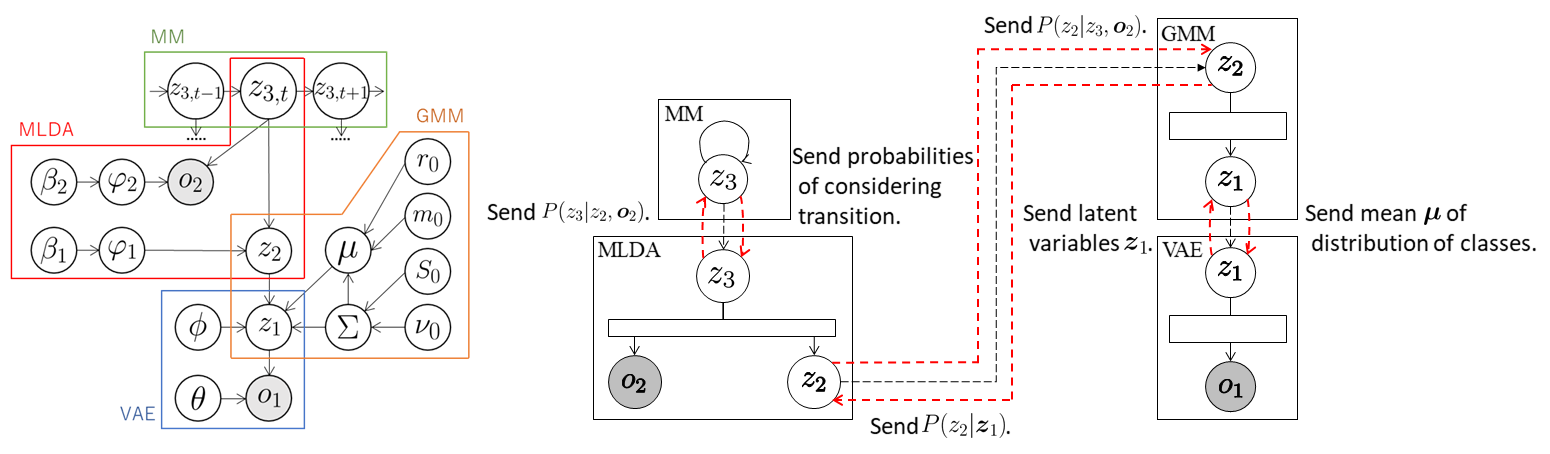## VAE+GMM+MLDA+MM

In this tutorial, we construct a model that classifies multimodal information considering a transition in an unsupervised manner by integrating VAE, GMM, MLDA, and MM.

### Data

We use a handwritten digit image dataset MNIST and a Spoken Arabic Digit Data Set. The spoken Arabic digit dataset consists of MFCC features obtained by converting spoken Arabic digits; the results are published in the UCI Machine Learning Repository. We generated multimodal data by pairing the images and speech samples, sorting them in ascending order, e.g., 0, 1, 2, 3, 4, 5, 6, 7, 8, 9, 0, $$\cdots$$, in order to learn the transition rules. The number of pairs used in this tutorial is 3,000. We use HAC features converted from MFCC features. See here for details of the HAC features.

### Model

The VAE compresses the observations $$\boldsymbol{o}_ 1$$ into arbitrary dimensional latent variables $$\boldsymbol{z}_ 1$$ through a neural network called the encoder and sends them to the GMM. The GMM classifies the latent variables $$\boldsymbol{z}_ 1$$ received from the VAE and sends the probabilities $$P(z_ {2,t} \mid \boldsymbol{z}_ {1,t})$$ that the t-th data element is classified into the class $$z_ {2,t}$$ to the MLDA. At the same time, the GMM sends the means $$\boldsymbol{\mu}$$ of the distributions of classes into which each data element is classified to the VAE. Therefore, the VAE learns the latent space suitable for the classification of the GMM by using $$\boldsymbol{\mu}$$. The MLDA handles $$z_ 2$$ as observations by sampling from the probabilities $$P(z_ {2,t} \mid \boldsymbol{z}_ {1,t})$$ received from the GMM and classifies $$z_ 2$$ and the observations $$\boldsymbol{o}_ 2$$. Next, it sends the probabilities $$P(z_ {3,t} \mid z_ {2,t}, \boldsymbol{o}_ {2,t})$$ to the MM and the probabilities $$P(z_ {2,t} \mid z_ {3,t}, \boldsymbol{o}_ {2,t})$$ to the GMM. The MM computes the probabilities that $$z_ 3$$ are classified into each class based on the transition probabilities and sends them to the MLDA. The MLDA classifies again using the received probabilities so that the classification is performed in consideration of the data transition. The GMM classifies again using the received probabilities $$P(z_ {2,t} \mid z_ {3,t}, \boldsymbol{o}_ {2,t})$$ so that the classification is performed considering $$z_ 3$$ and $$\boldsymbol{o}_ 2$$ under the influence of the MLDA.

Through the above procedure, the parameters are estimated based on their effects on each module.### Codes

First, the necessary modules are imported:

import serket as srk
import vae
import gmm
import mlda
import mm
import numpy as np


Next, data and correct labels are loaded. The data are sent as observations to the connected modules using srk.Observation:

obs1 = srk.Observation( np.loadtxt( "data1.txt" ) )  # image data
obs2 = srk.Observation( np.loadtxt( "data2.txt" ) )  # speech data


The modules VAE, GMM, MLDA, and MM used in the integrated model are then defined. In the VAE, the number of dimensions of the latent variables is 18, the number of epochs is 200, and the batch size is 500. In the GMM, the data are classified into ten classes. The optional argument data_category is a set of correct labels and used to compute classification accuracy. In the MLDA, the data are classified into ten classes using the weights [200,200] for the modalities. The optional argument data_category is a set of correct labels used to compute classification accuracy.

vae1 = vae.VAE( 18, itr=200, batch_size=500 )
gmm1 = gmm.GMM( 10, category=data_category )
mlda1 = mlda.MLDA( 10, [200,200], category=data_category )
mm1 = mm.MarkovModel()


The modules are then connected and the integrated model is constructed:

vae1.connect( obs1 )  # connect obs1 to vae1
gmm1.connect( vae1 )  # connect vae1 to gmm1
mlda1.connect( obs2, gmm1 )  # connect obs2 and gmm1 to mlda1
mm1.connect( mlda1 ) # connect mlda1 to mm1


Finally, the parameters of the whole model are learned by alternately updating the parameters of each module through exchanging messages:

for i in range(5):
vae1.update()  # train vae1
gmm1.update()  # train gmm1
mlda1.update()  # train mlda1
mm1.update()  # train mm1


### Result

If model training is successful, then the module002_vae, module003_gmm, module004_mlda, and module005_mm directories are created. The parameters of each module, probabilities, accuracy, and so on are stored in each directory. The results and accuracy of the classification are stored in module004_mlda. The indices of the classes into which each data element is classified are saved in class_learn.txt and the classification accuracy is saved in acc_learn.txt.